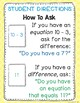# Subtraction Facts within 20 Go Fish GameSubject
Resource Type
File Type

PDF

(2 MB|39 pages)
Product Rating
Standards
Also included in:
1. ➯ Do you use Guided Math centers in your Kindergarten or First Grade classroom?➯ Do you want fun, engaging activities for your students to do INDEPENDENTLY?➯ Do you want accountability for your students?➯Do you want a great deal?!Then, this bundle is for you! This bundle includes:-10 separate Math G
\$37.50
\$25.00
Save \$12.50
• Product Description
• StandardsNEW

➯Then, this is the perfect component to a game center!

➯ A simple, yet fun math twist on the classic game of Go Fish!

Students start with 3 cards each. They ask a specific partner, if he or she has a match to their math fact.

For example, "Joey, do you have 7?" (to match his/her card "10 - 3"). Student answers Yes or GO FISH. If the first child gets a match, he/she gets to go again. Otherwise, students take turns asking for Subtraction Facts within 20 and THEIR MATCHES

This Resource Includes:

• Teacher Directions
• 2 versions of Student Directions
• Number Line to assist with adding
• 52 color Go Fish cards
• 52 black and white Go Fish cards
• Recording Sheet for student accountability
• Storage label
Fluently add and subtract within 20 using mental strategies. By end of Grade 2, know from memory all sums of two one-digit numbers.
Add and subtract within 20, demonstrating fluency for addition and subtraction within 10. Use strategies such as counting on; making ten (e.g., 8 + 6 = 8 + 2 + 4 = 10 + 4 = 14); decomposing a number leading to a ten (e.g., 13 - 4 = 13 - 3 - 1 = 10 - 1 = 9); using the relationship between addition and subtraction (e.g., knowing that 8 + 4 = 12, one knows 12 - 8 = 4); and creating equivalent but easier or known sums (e.g., adding 6 + 7 by creating the known equivalent 6 + 6 + 1 = 12 + 1 = 13).
Relate counting to addition and subtraction (e.g., by counting on 2 to add 2).
Understand subtraction as an unknown-addend problem. For example, subtract 10 – 8 by finding the number that makes 10 when added to 8.
Total Pages
39 pages
N/A
Teaching Duration
N/A
Report this Resource to TpT
Reported resources will be reviewed by our team. Report this resource to let us know if this resource violates TpT’s content guidelines.